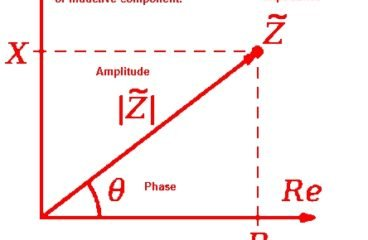;#### What is Impedance, Impedance vs Resistance

What is impedance Impedance (Z)  is similar to Resistance (R) . Impedance and Resistance both oppose the current in circuit. Both are almost the same thing , But resistance related to DC Circuit. Resistance oppose the steady electric current in DC circuit. Resistance remains same (constant) at any different frequency range. Impedance is related with AC circuit. Impedance vary […]